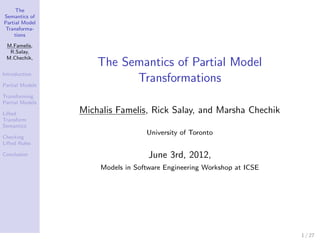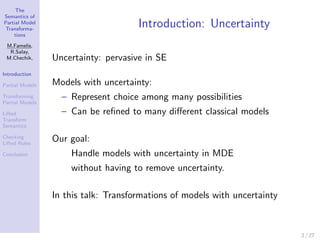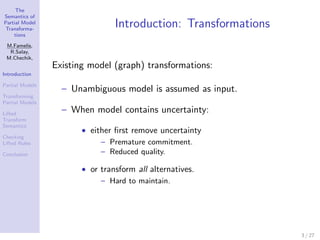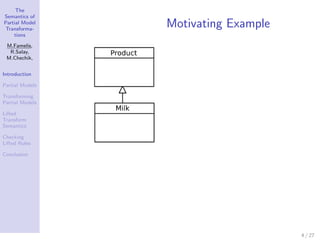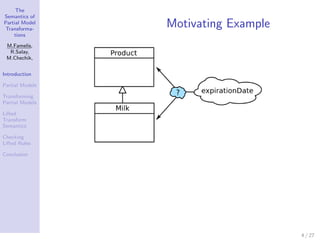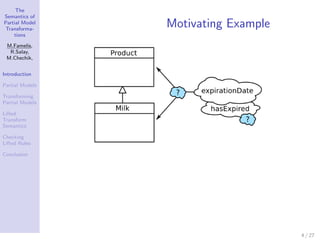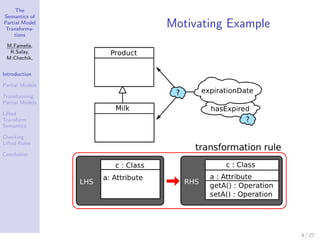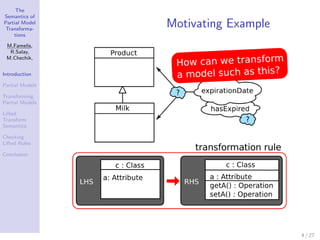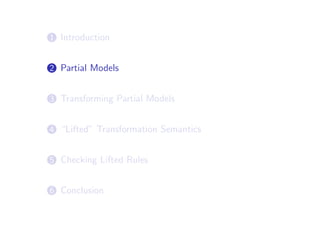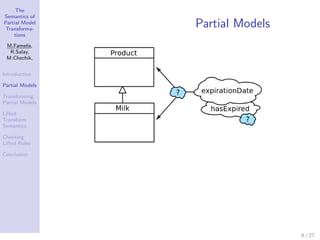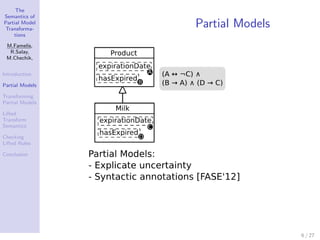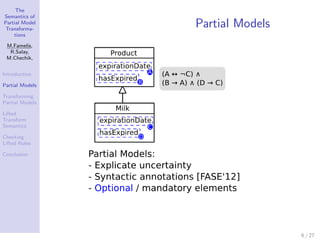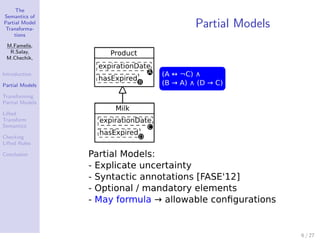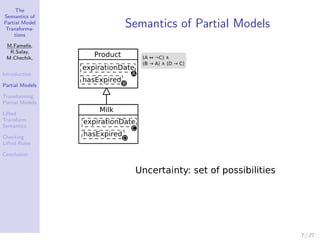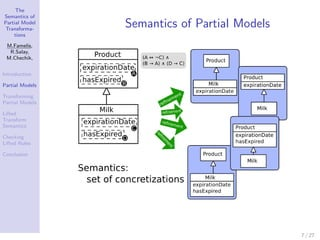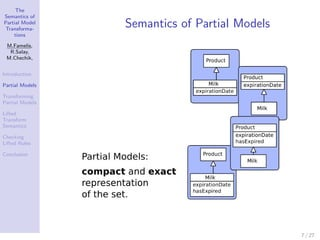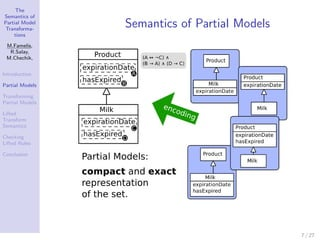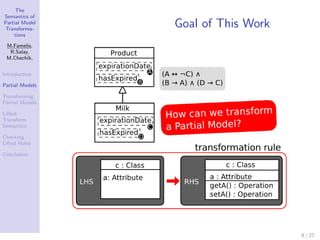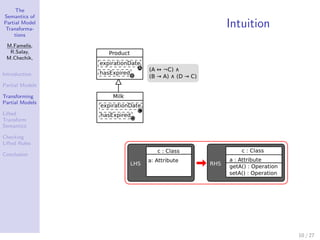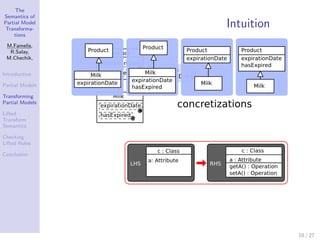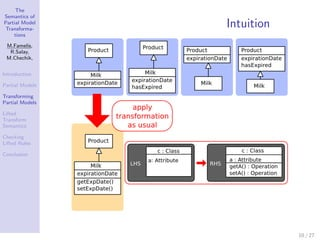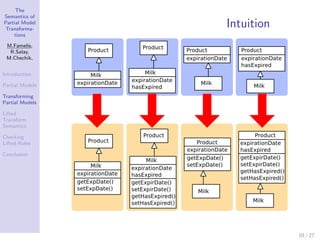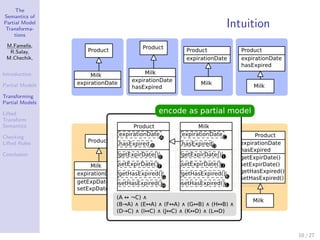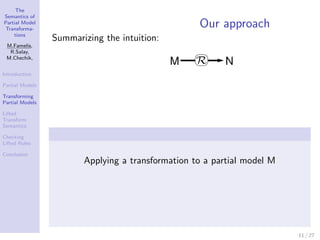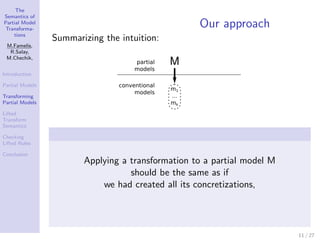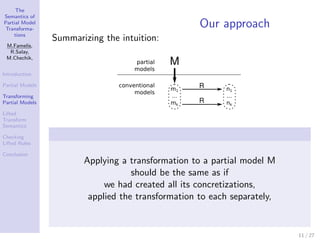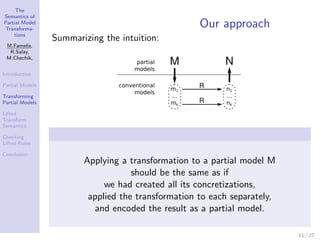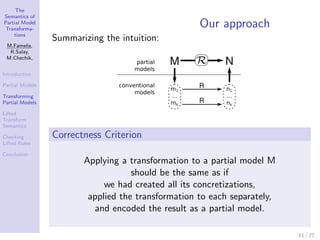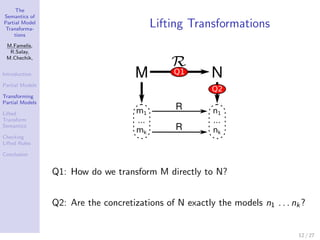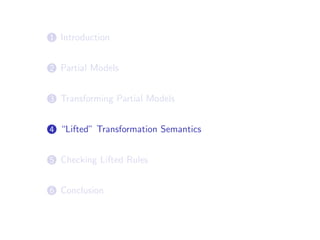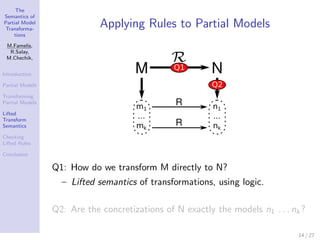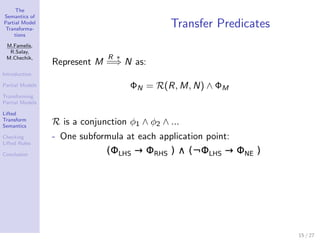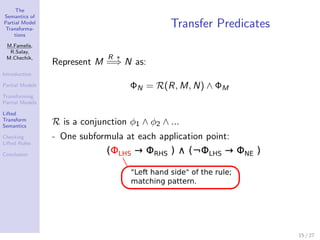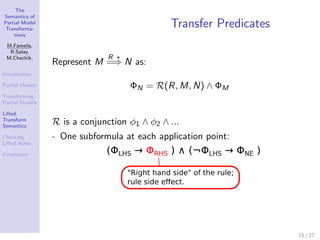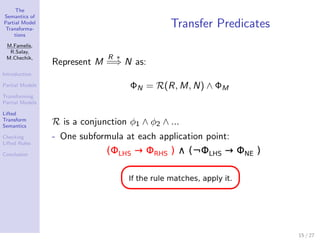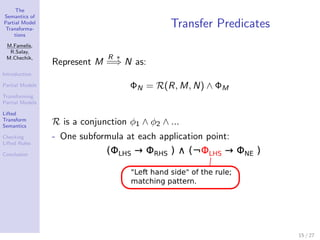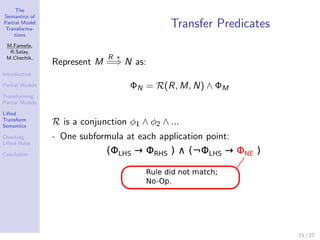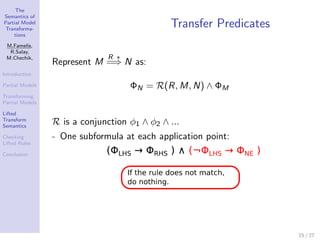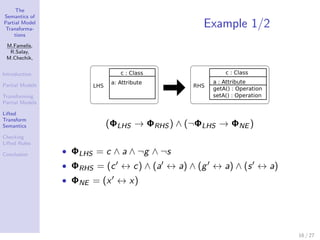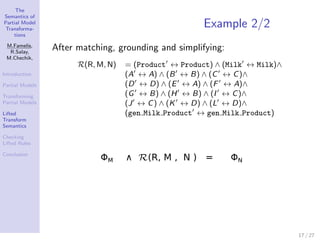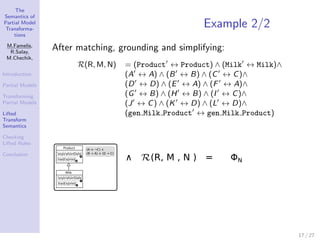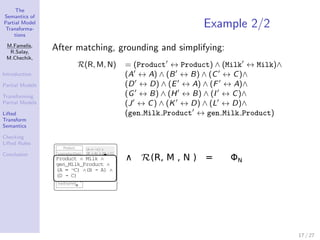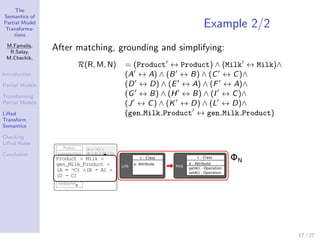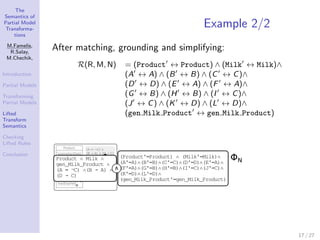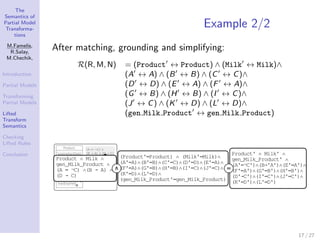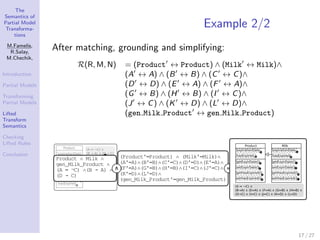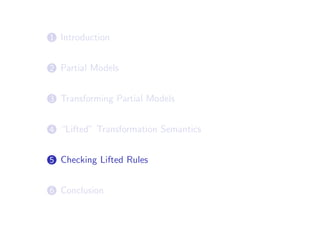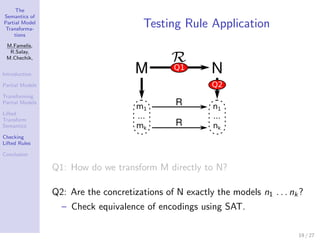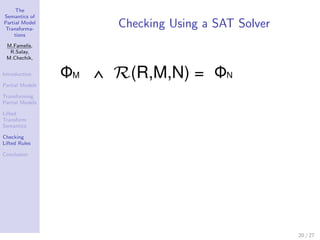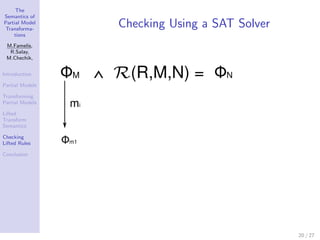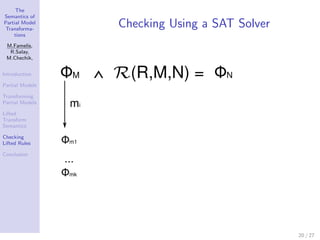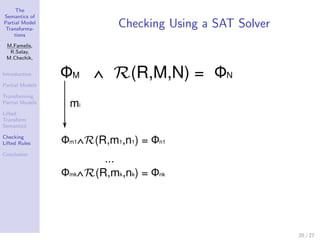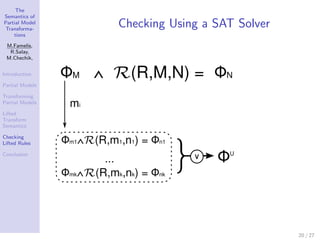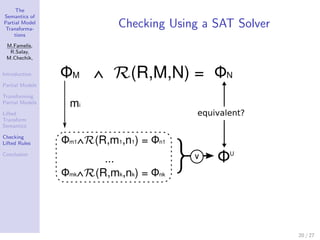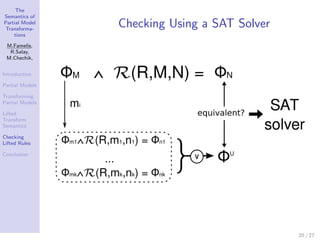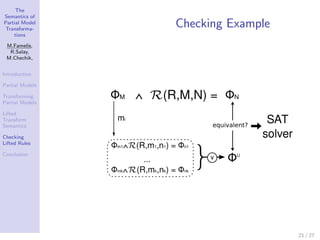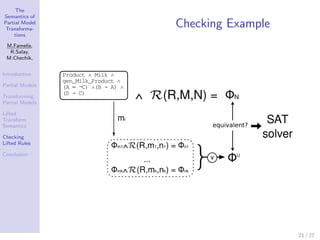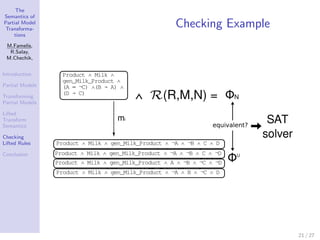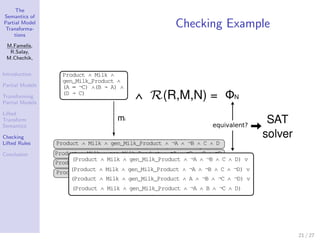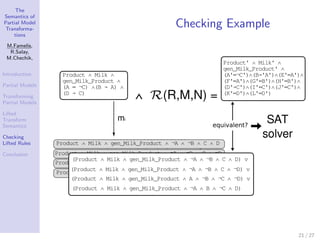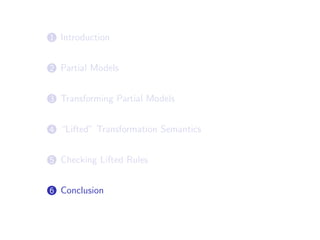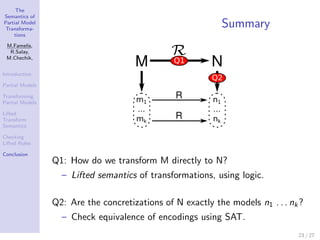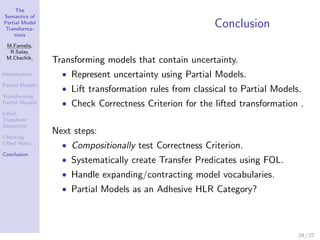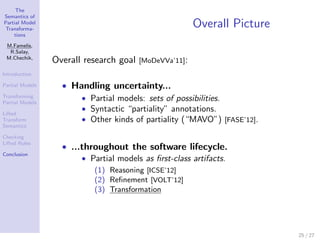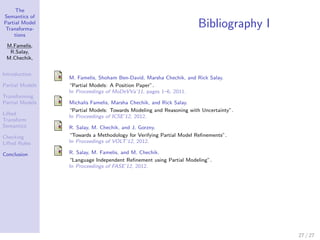1 of 67

## RecommendedUsing Developer Conversations to Resolve Uncertainty in Software Development:...Michalis FamelisTransformations of Models Containing UncertaintyMichalis FamelisDeveloping a new Epsilon EMC driverHoracio Hoyos RodríguezPartial Models: Towards Modeling and Reasoning with UncertaintyMichalis FamelisDeveloping a new Epsilon Language through Grammar Extension: The Epsilon Dem...Dimitris KolovosUncertainty Management With Partial ModelsMichalis Famelis

### The Semantics of Partial Model Transformations

1. The Semantics of Partial Model Transforma- tions M.Famelis, R.Salay, M.Chechik, The Semantics of Partial Model Introduction Partial Models Transformations Transforming Partial Models Lifted Michalis Famelis, Rick Salay, and Marsha Chechik Transform Semantics University of Toronto Checking Lifted Rules Conclusion June 3rd, 2012, Models in Software Engineering Workshop at ICSE 1 / 27
2. The Semantics of Partial Model Transforma- Introduction: Uncertainty tions M.Famelis, R.Salay, M.Chechik, Uncertainty: pervasive in SE Introduction Partial Models Models with uncertainty: Transforming Partial Models – Represent choice among many possibilities Lifted – Can be reﬁned to many diﬀerent classical models Transform Semantics Checking Lifted Rules Our goal: Conclusion Handle models with uncertainty in MDE without having to remove uncertainty. In this talk: Transformations of models with uncertainty 2 / 27
3. The Semantics of Partial Model Transforma- Introduction: Transformations tions M.Famelis, R.Salay, M.Chechik, Existing model (graph) transformations: Introduction Partial Models – Unambiguous model is assumed as input. Transforming Partial Models Lifted – When model contains uncertainty: Transform Semantics • either ﬁrst remove uncertainty Checking Lifted Rules – Premature commitment. Conclusion – Reduced quality. • or transform all alternatives. – Hard to maintain. 3 / 27
4. The Semantics of Partial Model Transforma- Motivating Example tions M.Famelis, R.Salay, M.Chechik, Introduction Partial Models Transforming Partial Models Lifted Transform Semantics Checking Lifted Rules Conclusion 4 / 27
5. The Semantics of Partial Model Transforma- Motivating Example tions M.Famelis, R.Salay, M.Chechik, Introduction Partial Models Transforming Partial Models Lifted Transform Semantics Checking Lifted Rules Conclusion 4 / 27
6. The Semantics of Partial Model Transforma- Motivating Example tions M.Famelis, R.Salay, M.Chechik, Introduction Partial Models Transforming Partial Models Lifted Transform Semantics Checking Lifted Rules Conclusion 4 / 27
7. The Semantics of Partial Model Transforma- Motivating Example tions M.Famelis, R.Salay, M.Chechik, Introduction Partial Models Transforming Partial Models Lifted Transform Semantics Checking Lifted Rules Conclusion 4 / 27
8. The Semantics of Partial Model Transforma- Motivating Example tions M.Famelis, R.Salay, M.Chechik, Introduction Partial Models Transforming Partial Models Lifted Transform Semantics Checking Lifted Rules Conclusion 4 / 27
9. 1 Introduction 2 Partial Models 3 Transforming Partial Models 4 “Lifted” Transformation Semantics 5 Checking Lifted Rules 6 Conclusion
10. The Semantics of Partial Model Transforma- Partial Models tions M.Famelis, R.Salay, M.Chechik, Introduction Partial Models Transforming Partial Models Lifted Transform Semantics Checking Lifted Rules Conclusion 6 / 27
11. The Semantics of Partial Model Transforma- Partial Models tions M.Famelis, R.Salay, M.Chechik, Introduction Partial Models Transforming Partial Models Lifted Transform Semantics Checking Lifted Rules Conclusion 6 / 27
12. The Semantics of Partial Model Transforma- Partial Models tions M.Famelis, R.Salay, M.Chechik, Introduction Partial Models Transforming Partial Models Lifted Transform Semantics Checking Lifted Rules Conclusion 6 / 27
13. The Semantics of Partial Model Transforma- Partial Models tions M.Famelis, R.Salay, M.Chechik, Introduction Partial Models Transforming Partial Models Lifted Transform Semantics Checking Lifted Rules Conclusion 6 / 27
14. The Semantics of Partial Model Transforma- Semantics of Partial Models tions M.Famelis, R.Salay, M.Chechik, Introduction Partial Models Transforming Partial Models Lifted Transform Semantics Checking Lifted Rules Conclusion 7 / 27
15. The Semantics of Partial Model Transforma- Semantics of Partial Models tions M.Famelis, R.Salay, M.Chechik, Introduction Partial Models Transforming Partial Models Lifted Transform Semantics Checking Lifted Rules Conclusion 7 / 27
16. The Semantics of Partial Model Transforma- Semantics of Partial Models tions M.Famelis, R.Salay, M.Chechik, Introduction Partial Models Transforming Partial Models Lifted Transform Semantics Checking Lifted Rules Conclusion 7 / 27
17. The Semantics of Partial Model Transforma- Semantics of Partial Models tions M.Famelis, R.Salay, M.Chechik, Introduction Partial Models Transforming Partial Models Lifted Transform Semantics Checking Lifted Rules Conclusion 7 / 27
18. The Semantics of Partial Model Transforma- Goal of This Work tions M.Famelis, R.Salay, M.Chechik, Introduction Partial Models Transforming Partial Models Lifted Transform Semantics Checking Lifted Rules Conclusion 8 / 27
19. 1 Introduction 2 Partial Models 3 Transforming Partial Models 4 “Lifted” Transformation Semantics 5 Checking Lifted Rules 6 Conclusion
20. The Semantics of Partial Model Transforma- Intuition tions M.Famelis, R.Salay, M.Chechik, Introduction Partial Models Transforming Partial Models Lifted Transform Semantics Checking Lifted Rules Conclusion 10 / 27
21. The Semantics of Partial Model Transforma- Intuition tions M.Famelis, R.Salay, M.Chechik, Introduction Partial Models Transforming Partial Models Lifted Transform Semantics Checking Lifted Rules Conclusion 10 / 27
22. The Semantics of Partial Model Transforma- Intuition tions M.Famelis, R.Salay, M.Chechik, Introduction Partial Models Transforming Partial Models Lifted Transform Semantics Checking Lifted Rules Conclusion 10 / 27
23. The Semantics of Partial Model Transforma- Intuition tions M.Famelis, R.Salay, M.Chechik, Introduction Partial Models Transforming Partial Models Lifted Transform Semantics Checking Lifted Rules Conclusion 10 / 27
24. The Semantics of Partial Model Transforma- Intuition tions M.Famelis, R.Salay, M.Chechik, Introduction Partial Models Transforming Partial Models Lifted Transform Semantics Checking Lifted Rules Conclusion 10 / 27
25. The Semantics of Partial Model Transforma- Our approach tions Summarizing the intuition: M.Famelis, R.Salay, M.Chechik, Introduction Partial Models Transforming Partial Models Lifted Transform Semantics Checking Lifted Rules Conclusion Applying a transformation to a partial model M 11 / 27
26. The Semantics of Partial Model Transforma- Our approach tions Summarizing the intuition: M.Famelis, R.Salay, M.Chechik, Introduction Partial Models Transforming Partial Models Lifted Transform Semantics Checking Lifted Rules Conclusion Applying a transformation to a partial model M should be the same as if we had created all its concretizations, 11 / 27
27. The Semantics of Partial Model Transforma- Our approach tions Summarizing the intuition: M.Famelis, R.Salay, M.Chechik, Introduction Partial Models Transforming Partial Models Lifted Transform Semantics Checking Lifted Rules Conclusion Applying a transformation to a partial model M should be the same as if we had created all its concretizations, applied the transformation to each separately, 11 / 27
28. The Semantics of Partial Model Transforma- Our approach tions Summarizing the intuition: M.Famelis, R.Salay, M.Chechik, Introduction Partial Models Transforming Partial Models Lifted Transform Semantics Checking Lifted Rules Conclusion Applying a transformation to a partial model M should be the same as if we had created all its concretizations, applied the transformation to each separately, and encoded the result as a partial model. 11 / 27
29. The Semantics of Partial Model Transforma- Our approach tions Summarizing the intuition: M.Famelis, R.Salay, M.Chechik, Introduction Partial Models Transforming Partial Models Lifted Transform Semantics Checking Correctness Criterion Lifted Rules Conclusion Applying a transformation to a partial model M should be the same as if we had created all its concretizations, applied the transformation to each separately, and encoded the result as a partial model. 11 / 27
30. The Semantics of Partial Model Transforma- Lifting Transformations tions M.Famelis, R.Salay, M.Chechik, Introduction Partial Models Transforming Partial Models Lifted Transform Semantics Checking Lifted Rules Conclusion Q1: How do we transform M directly to N? Q2: Are the concretizations of N exactly the models n1 . . . nk ? 12 / 27
31. 1 Introduction 2 Partial Models 3 Transforming Partial Models 4 “Lifted” Transformation Semantics 5 Checking Lifted Rules 6 Conclusion
32. The Semantics of Partial Model Transforma- Applying Rules to Partial Models tions M.Famelis, R.Salay, M.Chechik, Introduction Partial Models Transforming Partial Models Lifted Transform Semantics Checking Lifted Rules Conclusion Q1: How do we transform M directly to N? – Lifted semantics of transformations, using logic. Q2: Are the concretizations of N exactly the models n1 . . . nk ? 14 / 27
33. The Semantics of Partial Model Transforma- Transfer Predicates tions M.Famelis, R.Salay, M.Chechik, R ∗ Represent M =⇒ N as: Introduction Partial Models ΦN = R(R, M, N) ∧ ΦM Transforming Partial Models Lifted Transform Semantics R is a conjunction φ1 ∧ φ2 ∧ ... Checking - One subformula at each application point: Lifted Rules Conclusion 15 / 27
34. The Semantics of Partial Model Transforma- Transfer Predicates tions M.Famelis, R.Salay, M.Chechik, R ∗ Represent M =⇒ N as: Introduction Partial Models ΦN = R(R, M, N) ∧ ΦM Transforming Partial Models Lifted Transform Semantics R is a conjunction φ1 ∧ φ2 ∧ ... Checking - One subformula at each application point: Lifted Rules Conclusion 15 / 27
35. The Semantics of Partial Model Transforma- Transfer Predicates tions M.Famelis, R.Salay, M.Chechik, R ∗ Represent M =⇒ N as: Introduction Partial Models ΦN = R(R, M, N) ∧ ΦM Transforming Partial Models Lifted Transform Semantics R is a conjunction φ1 ∧ φ2 ∧ ... Checking - One subformula at each application point: Lifted Rules Conclusion 15 / 27
36. The Semantics of Partial Model Transforma- Transfer Predicates tions M.Famelis, R.Salay, M.Chechik, R ∗ Represent M =⇒ N as: Introduction Partial Models ΦN = R(R, M, N) ∧ ΦM Transforming Partial Models Lifted Transform Semantics R is a conjunction φ1 ∧ φ2 ∧ ... Checking - One subformula at each application point: Lifted Rules Conclusion 15 / 27
37. The Semantics of Partial Model Transforma- Transfer Predicates tions M.Famelis, R.Salay, M.Chechik, R ∗ Represent M =⇒ N as: Introduction Partial Models ΦN = R(R, M, N) ∧ ΦM Transforming Partial Models Lifted Transform Semantics R is a conjunction φ1 ∧ φ2 ∧ ... Checking - One subformula at each application point: Lifted Rules Conclusion 15 / 27
38. The Semantics of Partial Model Transforma- Transfer Predicates tions M.Famelis, R.Salay, M.Chechik, R ∗ Represent M =⇒ N as: Introduction Partial Models ΦN = R(R, M, N) ∧ ΦM Transforming Partial Models Lifted Transform Semantics R is a conjunction φ1 ∧ φ2 ∧ ... Checking - One subformula at each application point: Lifted Rules Conclusion 15 / 27
39. The Semantics of Partial Model Transforma- Transfer Predicates tions M.Famelis, R.Salay, M.Chechik, R ∗ Represent M =⇒ N as: Introduction Partial Models ΦN = R(R, M, N) ∧ ΦM Transforming Partial Models Lifted Transform Semantics R is a conjunction φ1 ∧ φ2 ∧ ... Checking - One subformula at each application point: Lifted Rules Conclusion 15 / 27
40. The Semantics of Partial Model Transforma- Example 1/2 tions M.Famelis, R.Salay, M.Chechik, Introduction Partial Models Transforming Partial Models Lifted Transform Semantics (ΦLHS → ΦRHS ) ∧ (¬ΦLHS → ΦNE ) Checking Lifted Rules Conclusion • ΦLHS = c ∧ a ∧ ¬g ∧ ¬s • ΦRHS = (c ↔ c) ∧ (a ↔ a) ∧ (g ↔ a) ∧ (s ↔ a) • ΦNE = (x ↔ x) 16 / 27
41. The Semantics of Partial Model Transforma- Example 2/2 tions M.Famelis, R.Salay, After matching, grounding and simplifying: M.Chechik, R(R, M, N) = (Product ↔ Product) ∧ (Milk ↔ Milk)∧ Introduction (A ↔ A) ∧ (B ↔ B) ∧ (C ↔ C )∧ Partial Models (D ↔ D) ∧ (E ↔ A) ∧ (F ↔ A)∧ Transforming (G ↔ B) ∧ (H ↔ B) ∧ (I ↔ C )∧ Partial Models (J ↔ C ) ∧ (K ↔ D) ∧ (L ↔ D)∧ Lifted (gen Milk Product ↔ gen Milk Product) Transform Semantics Checking Lifted Rules Conclusion 17 / 27
42. The Semantics of Partial Model Transforma- Example 2/2 tions M.Famelis, R.Salay, After matching, grounding and simplifying: M.Chechik, R(R, M, N) = (Product ↔ Product) ∧ (Milk ↔ Milk)∧ Introduction (A ↔ A) ∧ (B ↔ B) ∧ (C ↔ C )∧ Partial Models (D ↔ D) ∧ (E ↔ A) ∧ (F ↔ A)∧ Transforming (G ↔ B) ∧ (H ↔ B) ∧ (I ↔ C )∧ Partial Models (J ↔ C ) ∧ (K ↔ D) ∧ (L ↔ D)∧ Lifted (gen Milk Product ↔ gen Milk Product) Transform Semantics Checking Lifted Rules Conclusion 17 / 27
43. The Semantics of Partial Model Transforma- Example 2/2 tions M.Famelis, R.Salay, After matching, grounding and simplifying: M.Chechik, R(R, M, N) = (Product ↔ Product) ∧ (Milk ↔ Milk)∧ Introduction (A ↔ A) ∧ (B ↔ B) ∧ (C ↔ C )∧ Partial Models (D ↔ D) ∧ (E ↔ A) ∧ (F ↔ A)∧ Transforming (G ↔ B) ∧ (H ↔ B) ∧ (I ↔ C )∧ Partial Models (J ↔ C ) ∧ (K ↔ D) ∧ (L ↔ D)∧ Lifted (gen Milk Product ↔ gen Milk Product) Transform Semantics Checking Lifted Rules Conclusion 17 / 27
44. The Semantics of Partial Model Transforma- Example 2/2 tions M.Famelis, R.Salay, After matching, grounding and simplifying: M.Chechik, R(R, M, N) = (Product ↔ Product) ∧ (Milk ↔ Milk)∧ Introduction (A ↔ A) ∧ (B ↔ B) ∧ (C ↔ C )∧ Partial Models (D ↔ D) ∧ (E ↔ A) ∧ (F ↔ A)∧ Transforming (G ↔ B) ∧ (H ↔ B) ∧ (I ↔ C )∧ Partial Models (J ↔ C ) ∧ (K ↔ D) ∧ (L ↔ D)∧ Lifted (gen Milk Product ↔ gen Milk Product) Transform Semantics Checking Lifted Rules Conclusion 17 / 27
45. The Semantics of Partial Model Transforma- Example 2/2 tions M.Famelis, R.Salay, After matching, grounding and simplifying: M.Chechik, R(R, M, N) = (Product ↔ Product) ∧ (Milk ↔ Milk)∧ Introduction (A ↔ A) ∧ (B ↔ B) ∧ (C ↔ C )∧ Partial Models (D ↔ D) ∧ (E ↔ A) ∧ (F ↔ A)∧ Transforming (G ↔ B) ∧ (H ↔ B) ∧ (I ↔ C )∧ Partial Models (J ↔ C ) ∧ (K ↔ D) ∧ (L ↔ D)∧ Lifted (gen Milk Product ↔ gen Milk Product) Transform Semantics Checking Lifted Rules Conclusion 17 / 27
46. The Semantics of Partial Model Transforma- Example 2/2 tions M.Famelis, R.Salay, After matching, grounding and simplifying: M.Chechik, R(R, M, N) = (Product ↔ Product) ∧ (Milk ↔ Milk)∧ Introduction (A ↔ A) ∧ (B ↔ B) ∧ (C ↔ C )∧ Partial Models (D ↔ D) ∧ (E ↔ A) ∧ (F ↔ A)∧ Transforming (G ↔ B) ∧ (H ↔ B) ∧ (I ↔ C )∧ Partial Models (J ↔ C ) ∧ (K ↔ D) ∧ (L ↔ D)∧ Lifted (gen Milk Product ↔ gen Milk Product) Transform Semantics Checking Lifted Rules Conclusion 17 / 27
47. The Semantics of Partial Model Transforma- Example 2/2 tions M.Famelis, R.Salay, After matching, grounding and simplifying: M.Chechik, R(R, M, N) = (Product ↔ Product) ∧ (Milk ↔ Milk)∧ Introduction (A ↔ A) ∧ (B ↔ B) ∧ (C ↔ C )∧ Partial Models (D ↔ D) ∧ (E ↔ A) ∧ (F ↔ A)∧ Transforming (G ↔ B) ∧ (H ↔ B) ∧ (I ↔ C )∧ Partial Models (J ↔ C ) ∧ (K ↔ D) ∧ (L ↔ D)∧ Lifted (gen Milk Product ↔ gen Milk Product) Transform Semantics Checking Lifted Rules Conclusion 17 / 27
48. 1 Introduction 2 Partial Models 3 Transforming Partial Models 4 “Lifted” Transformation Semantics 5 Checking Lifted Rules 6 Conclusion
49. The Semantics of Partial Model Transforma- Testing Rule Application tions M.Famelis, R.Salay, M.Chechik, Introduction Partial Models Transforming Partial Models Lifted Transform Semantics Checking Lifted Rules Conclusion Q1: How do we transform M directly to N? Q2: Are the concretizations of N exactly the models n1 . . . nk ? – Check equivalence of encodings using SAT. 19 / 27
50. The Semantics of Partial Model Transforma- Checking Using a SAT Solver tions M.Famelis, R.Salay, M.Chechik, Introduction Partial Models Transforming Partial Models Lifted Transform Semantics Checking Lifted Rules Conclusion 20 / 27
51. The Semantics of Partial Model Transforma- Checking Using a SAT Solver tions M.Famelis, R.Salay, M.Chechik, Introduction Partial Models Transforming Partial Models Lifted Transform Semantics Checking Lifted Rules Conclusion 20 / 27
52. The Semantics of Partial Model Transforma- Checking Using a SAT Solver tions M.Famelis, R.Salay, M.Chechik, Introduction Partial Models Transforming Partial Models Lifted Transform Semantics Checking Lifted Rules Conclusion 20 / 27
53. The Semantics of Partial Model Transforma- Checking Using a SAT Solver tions M.Famelis, R.Salay, M.Chechik, Introduction Partial Models Transforming Partial Models Lifted Transform Semantics Checking Lifted Rules Conclusion 20 / 27
54. The Semantics of Partial Model Transforma- Checking Using a SAT Solver tions M.Famelis, R.Salay, M.Chechik, Introduction Partial Models Transforming Partial Models Lifted Transform Semantics Checking Lifted Rules Conclusion 20 / 27
55. The Semantics of Partial Model Transforma- Checking Using a SAT Solver tions M.Famelis, R.Salay, M.Chechik, Introduction Partial Models Transforming Partial Models Lifted Transform Semantics Checking Lifted Rules Conclusion 20 / 27
56. The Semantics of Partial Model Transforma- Checking Using a SAT Solver tions M.Famelis, R.Salay, M.Chechik, Introduction Partial Models Transforming Partial Models Lifted Transform Semantics Checking Lifted Rules Conclusion 20 / 27
57. The Semantics of Partial Model Transforma- Checking Example tions M.Famelis, R.Salay, M.Chechik, Introduction Partial Models Transforming Partial Models Lifted Transform Semantics Checking Lifted Rules Conclusion 21 / 27
58. The Semantics of Partial Model Transforma- Checking Example tions M.Famelis, R.Salay, M.Chechik, Introduction Partial Models Transforming Partial Models Lifted Transform Semantics Checking Lifted Rules Conclusion 21 / 27
59. The Semantics of Partial Model Transforma- Checking Example tions M.Famelis, R.Salay, M.Chechik, Introduction Partial Models Transforming Partial Models Lifted Transform Semantics Checking Lifted Rules Conclusion 21 / 27
60. The Semantics of Partial Model Transforma- Checking Example tions M.Famelis, R.Salay, M.Chechik, Introduction Partial Models Transforming Partial Models Lifted Transform Semantics Checking Lifted Rules Conclusion 21 / 27
61. The Semantics of Partial Model Transforma- Checking Example tions M.Famelis, R.Salay, M.Chechik, Introduction Partial Models Transforming Partial Models Lifted Transform Semantics Checking Lifted Rules Conclusion 21 / 27
62. 1 Introduction 2 Partial Models 3 Transforming Partial Models 4 “Lifted” Transformation Semantics 5 Checking Lifted Rules 6 Conclusion
63. The Semantics of Partial Model Transforma- Summary tions M.Famelis, R.Salay, M.Chechik, Introduction Partial Models Transforming Partial Models Lifted Transform Semantics Checking Lifted Rules Conclusion Q1: How do we transform M directly to N? – Lifted semantics of transformations, using logic. Q2: Are the concretizations of N exactly the models n1 . . . nk ? – Check equivalence of encodings using SAT. 23 / 27
64. The Semantics of Partial Model Transforma- Conclusion tions M.Famelis, R.Salay, M.Chechik, Transforming models that contain uncertainty. Introduction • Represent uncertainty using Partial Models. Partial Models • Lift transformation rules from classical to Partial Models. Transforming Partial Models • Check Correctness Criterion for the lifted transformation . Lifted Transform Semantics Next steps: Checking Lifted Rules • Compositionally test Correctness Criterion. Conclusion • Systematically create Transfer Predicates using FOL. • Handle expanding/contracting model vocabularies. • Partial Models as an Adhesive HLR Category? 24 / 27
65. The Semantics of Partial Model Transforma- Overall Picture tions M.Famelis, R.Salay, M.Chechik, Overall research goal [MoDeVVa’11]: Introduction Partial Models • Handling uncertainty... Transforming • Partial models: sets of possibilities. Partial Models • Syntactic “partiality” annotations. Lifted Transform • Other kinds of partiality (“MAVO”) [FASE’12]. Semantics Checking Lifted Rules • ...throughout the software lifecycle. Conclusion • Partial models as ﬁrst-class artifacts. (1) Reasoning [ICSE’12] (2) Reﬁnement [VOLT’12] (3) Transformation 25 / 27
66. Questions?
67. The Semantics of Partial Model Transforma- Bibliography I tions M.Famelis, R.Salay, M.Chechik, Introduction M. Famelis, Shoham Ben-David, Marsha Chechik, and Rick Salay. Partial Models “Partial Models: A Position Paper”. In Proceedings of MoDeVVa’11, pages 1–6, 2011. Transforming Partial Models Michalis Famelis, Marsha Chechik, and Rick Salay. “Partial Models: Towards Modeling and Reasoning with Uncertainty”. Lifted In Proceedings of ICSE’12, 2012. Transform Semantics R. Salay, M. Chechik, and J. Gorzny. Checking “Towards a Methodology for Verifying Partial Model Reﬁnements”. Lifted Rules In Proceedings of VOLT’12, 2012. Conclusion R. Salay, M. Famelis, and M. Chechik. “Language Independent Reﬁnement using Partial Modeling”. In Proceedings of FASE’12, 2012. 27 / 27